# Visibilities and Staticness Aaron Bloomfield CS 101 E

• Slides: 55
Download presentationVisibilities and Static-ness Aaron Bloomfield CS 101 -E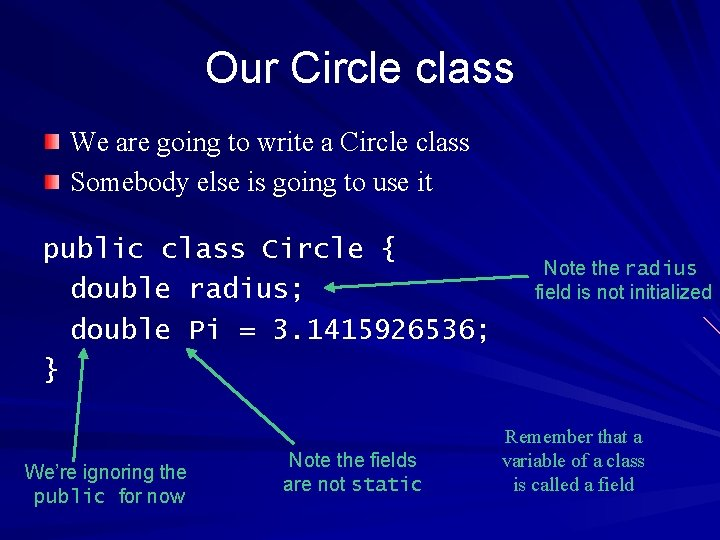Our Circle class We are going to write a Circle class Somebody else is going to use it public class Circle { double radius; double Pi = 3. 1415926536; } We’re ignoring the public for now Note the fields are not static Note the radius field is not initialized Remember that a variable of a class is called a field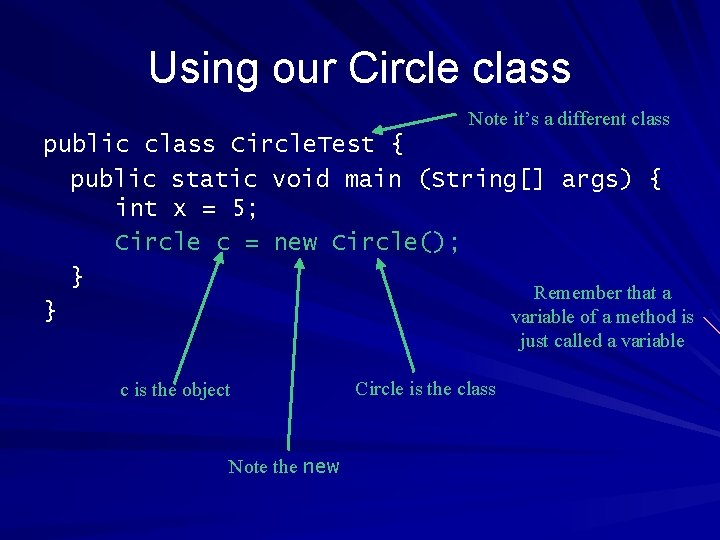Using our Circle class Note it’s a different class public class Circle. Test { public static void main (String[] args) { int x = 5; Circle c = new Circle(); } Remember that a } variable of a method is just called a variable c is the object Note the new Circle is the class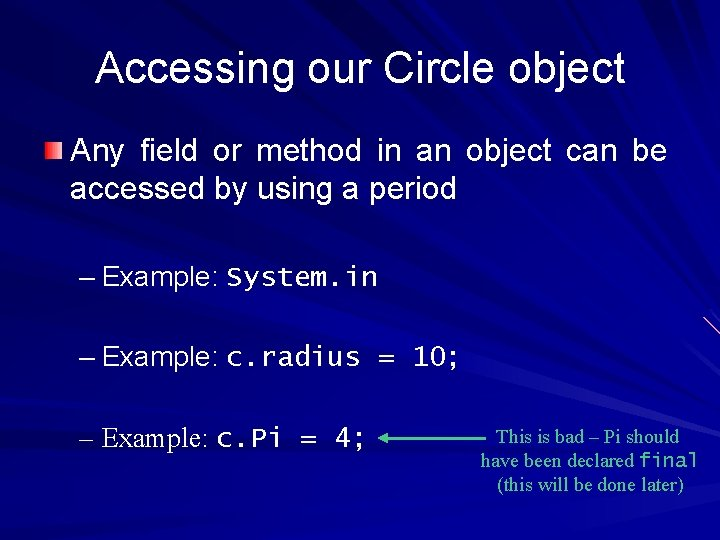Accessing our Circle object Any field or method in an object can be accessed by using a period – Example: System. in – Example: c. radius = 10; – Example: c. Pi = 4; This is bad – Pi should have been declared final (this will be done later)What’s the output? public class Circle { double radius; double Pi = 3. 1415926536; } public class Circle. Test { public static void main (String[] args) { int x; Circle c = new Circle(); Java will give a System. out. println (x); “variable not } initialized” error }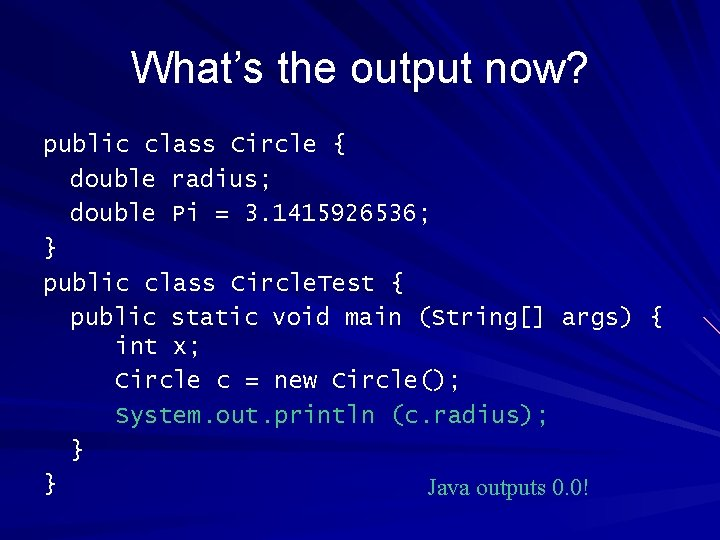What’s the output now? public class Circle { double radius; double Pi = 3. 1415926536; } public class Circle. Test { public static void main (String[] args) { int x; Circle c = new Circle(); System. out. println (c. radius); } } Java outputs 0. 0!What’s going on? A (method) variable needs to be initialized before it is used A field (class variable) is automatically initialized by Java – All doubles are initialized to 0. 0, ints to 0, etc. This is a bit counter-intuitive…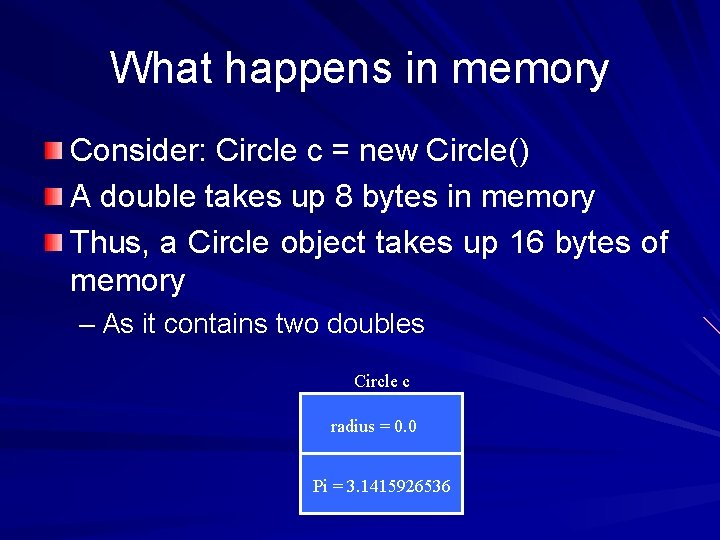What happens in memory Consider: Circle c = new Circle() A double takes up 8 bytes in memory Thus, a Circle object takes up 16 bytes of memory – As it contains two doubles Circle c radius = 0. 0 Pi = 3. 1415926536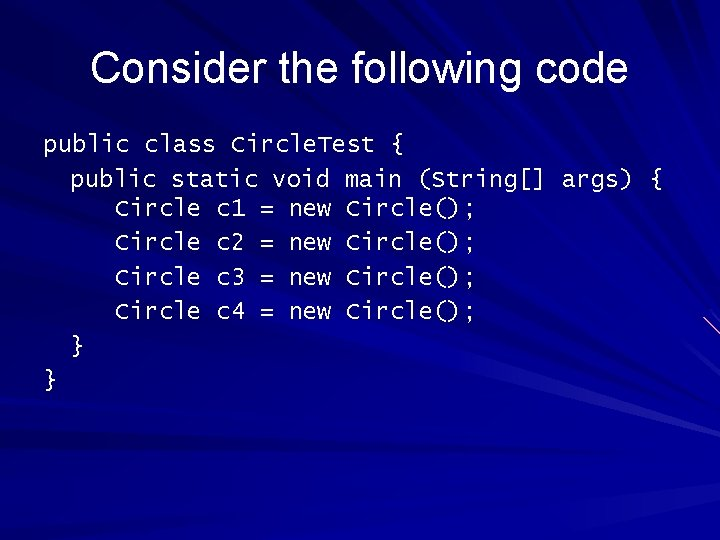Consider the following code public class Circle. Test { public static void main (String[] args) { Circle c 1 = new Circle(); Circle c 2 = new Circle(); Circle c 3 = new Circle(); Circle c 4 = new Circle(); } }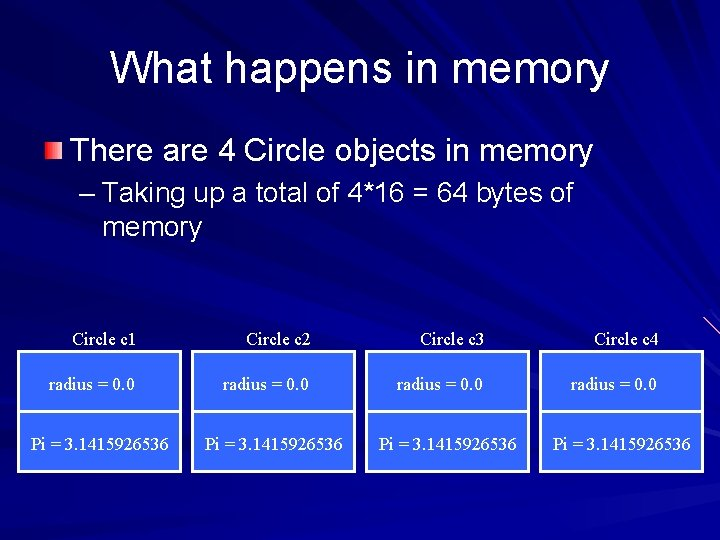What happens in memory There are 4 Circle objects in memory – Taking up a total of 4*16 = 64 bytes of memory Circle c 1 Circle c 2 Circle c 3 Circle c 4 radius = 0. 0 Pi = 3. 1415926536Consider the following code public class Circle. Test { public static void main (String[] args) { Circle c 1 = new Circle(); //. . . Circle c 1000000 = new Circle(); } } This program creates 1 million Circle objects!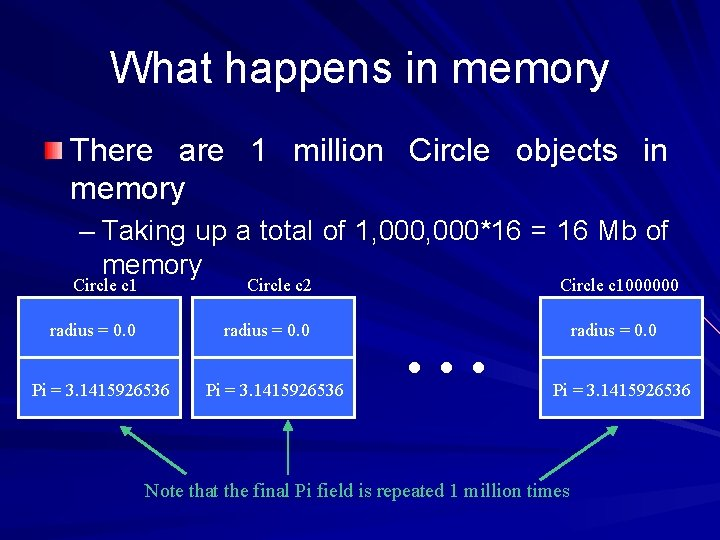What happens in memory There are 1 million Circle objects in memory – Taking up a total of 1, 000*16 = 16 Mb of memory Circle c 1 Circle c 2 radius = 0. 0 Pi = 3. 1415926536 … Circle c 1000000 radius = 0. 0 Pi = 3. 1415926536 Note that the final Pi field is repeated 1 million times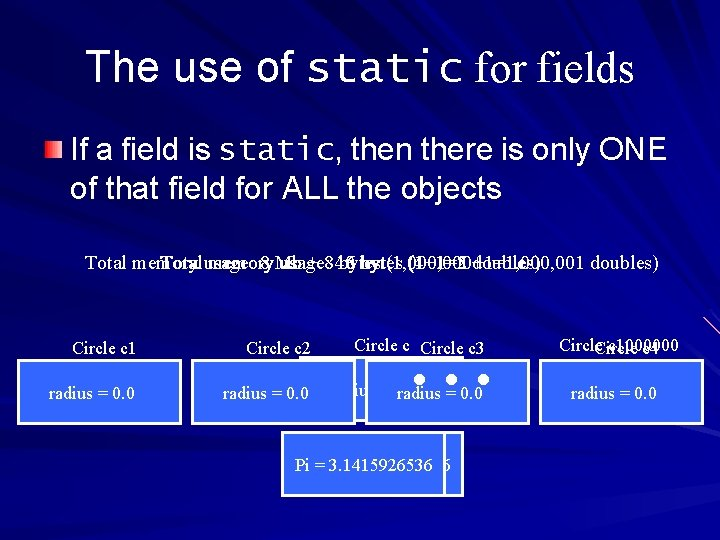The use of static for fields If a field is static, then there is only ONE of that field for ALL the objects 16 (1+1=2 doubles) Total memory Total usage: memory 8 Mb usage: + 8 40 bytes (1, 000+1=1, 000, 001 (4+1=5 doubles) … Circle c 1 Circle c 2 Circle c 3 radius = 0. 0 Pi Pi = 3. 1415926536 Circle c 1000000 Circle c 4 radius = 0. 0More on static fields What does the following print – Note that Pi is not final Circle c 1 = new Circle(); Circle c 2 = new Circle(); Circle c 3 = new Circle(); Circle c 4 = new Circle(); c 1. Pi = 4. 3; System. out. println (c 2. Pi); Note you can refer to static fields by object. field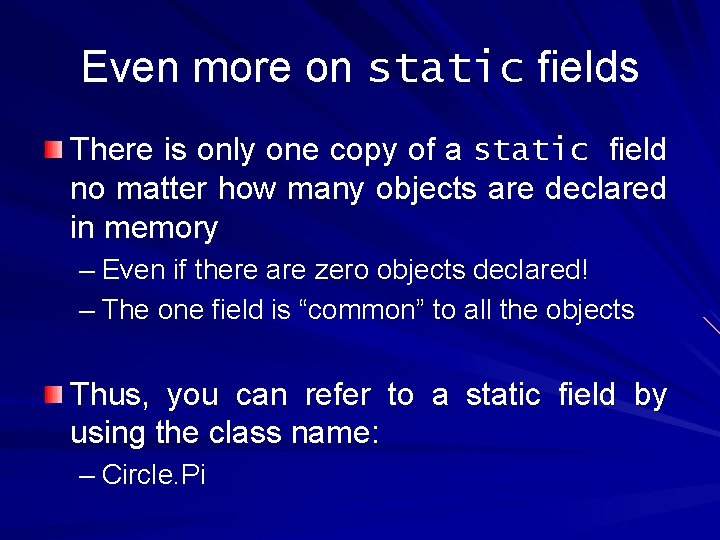Even more on static fields There is only one copy of a static field no matter how many objects are declared in memory – Even if there are zero objects declared! – The one field is “common” to all the objects Thus, you can refer to a static field by using the class name: – Circle. Pi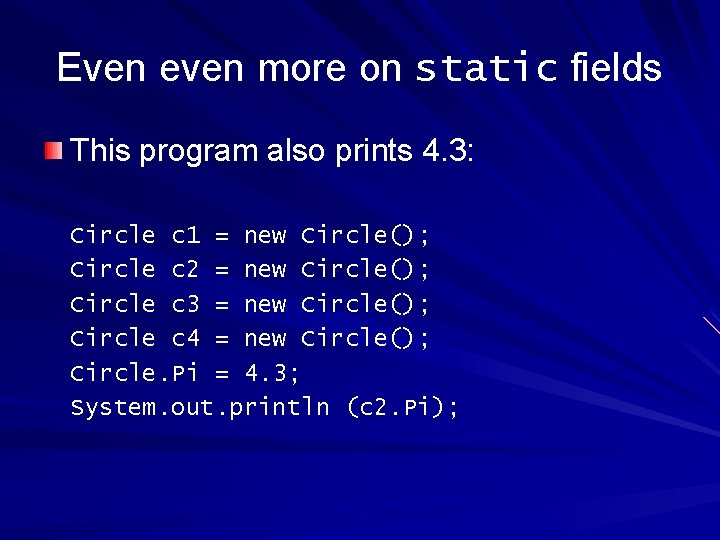Even even more on static fields This program also prints 4. 3: Circle c 1 = new Circle(); Circle c 2 = new Circle(); Circle c 3 = new Circle(); Circle c 4 = new Circle(); Circle. Pi = 4. 3; System. out. println (c 2. Pi);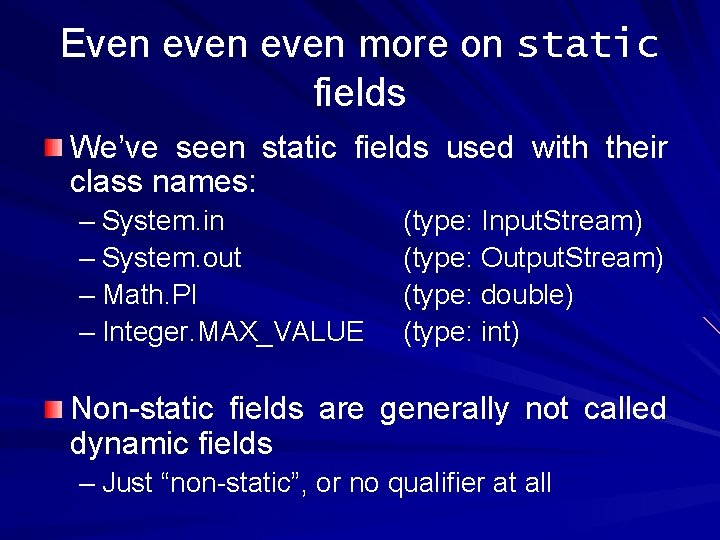Even even more on static fields We’ve seen static fields used with their class names: – System. in – System. out – Math. PI – Integer. MAX_VALUE (type: Input. Stream) (type: Output. Stream) (type: double) (type: int) Non-static fields are generally not called dynamic fields – Just “non-static”, or no qualifier at allA bit of humor…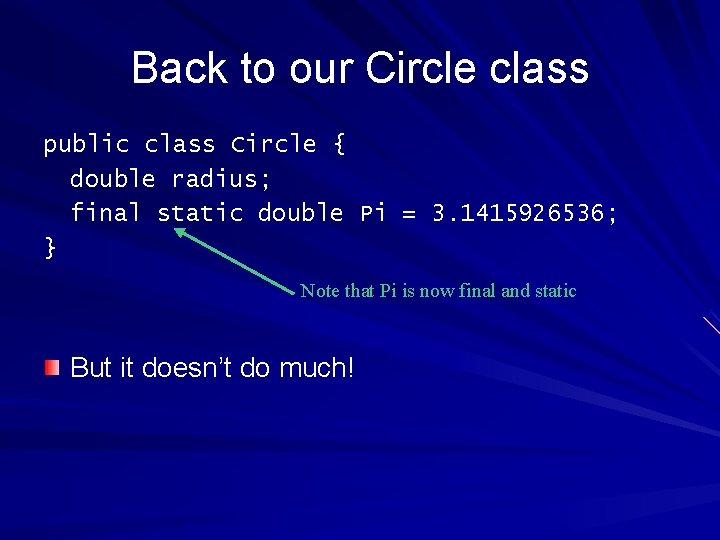Back to our Circle class public class Circle { double radius; final static double Pi = 3. 1415926536; } Note that Pi is now final and static But it doesn’t do much!Adding a method public class Circle { double radius; final static double Pi = 3. 1415926536; double compute. Area () { return Pi*radius; } } Note that a (non-static) method can use all the fields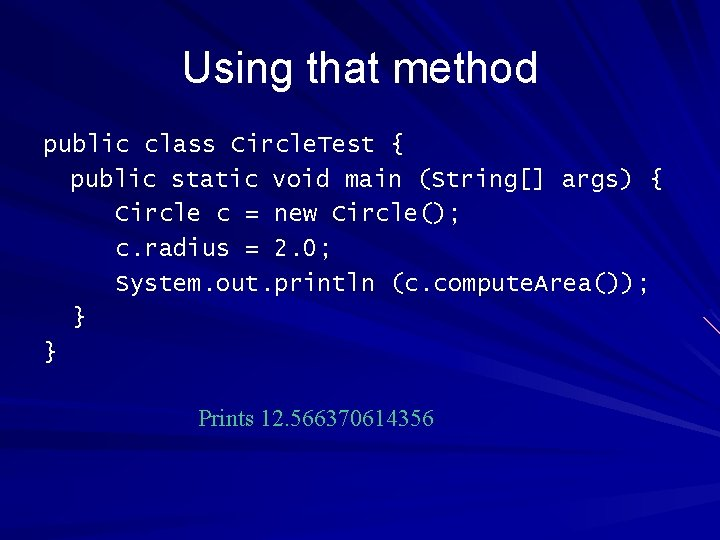Using that method public class Circle. Test { public static void main (String[] args) { Circle c = new Circle(); c. radius = 2. 0; System. out. println (c. compute. Area()); } } Prints 12. 566370614356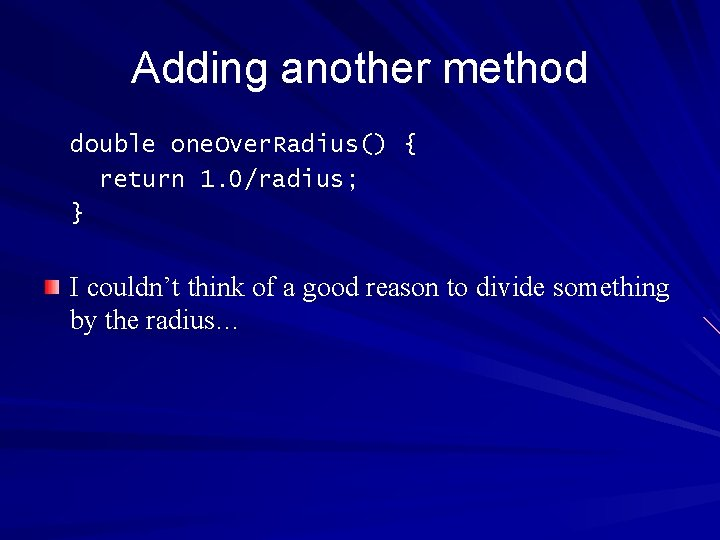Adding another method double one. Over. Radius() { return 1. 0/radius; } I couldn’t think of a good reason to divide something by the radius…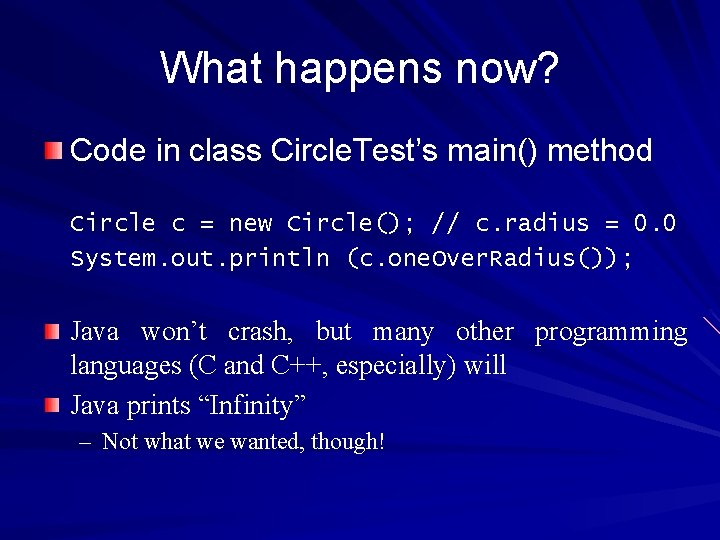What happens now? Code in class Circle. Test’s main() method Circle c = new Circle(); // c. radius = 0. 0 System. out. println (c. one. Over. Radius()); Java won’t crash, but many other programming languages (C and C++, especially) will Java prints “Infinity” – Not what we wanted, though!One way to fix this… Note that the radius field is now initialized to 1. 0 public class Circle { double radius = 1. 0; final static double Pi = 3. 1415926536; double compute. Area () { return Pi*radius; } double one. Over. Radius() { return 1. 0/radius; } }Back to our program… This code will now run properly: Circle c = new Circle(); // c. radius = 1. 0 System. out. println (c. one. Over. Radius()); But this code will “crash”: Circle c = new Circle(); // c. radius = 1. 0 c. radius = 0. 0; System. out. println (c. one. Over. Radius());Where the “crash” occurs public class Circle. Test { public static void main (String[] args) { Circle c = new Circle(); // c. radius = 1. 0 c. radius = 0. 0; System. out. println (c. one. Over. Radius()); } } Here is the badly written code public class Circle { double radius = 1. 0; final static double Pi = 3. 1415926536; double compute. Area () { return Pi*radius; } double one. Over. Radius() { return 1. 0/radius; } Here is where the “crash” occursMotivation for private fields Problem: We do not want people using our Circle class to be able to modify the fields on their own Solution: Don’t allow other code to modify the radius field – Give it private visibility private means that only code within the class can modify the field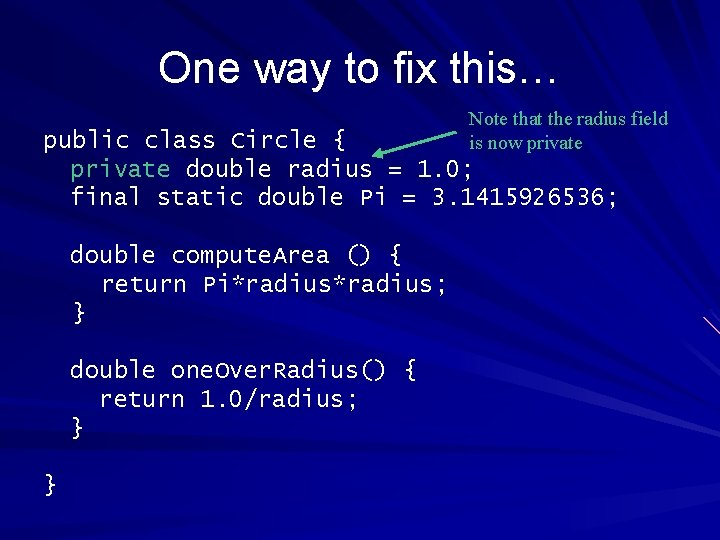One way to fix this… Note that the radius field is now private public class Circle { private double radius = 1. 0; final static double Pi = 3. 1415926536; double compute. Area () { return Pi*radius; } double one. Over. Radius() { return 1. 0/radius; } }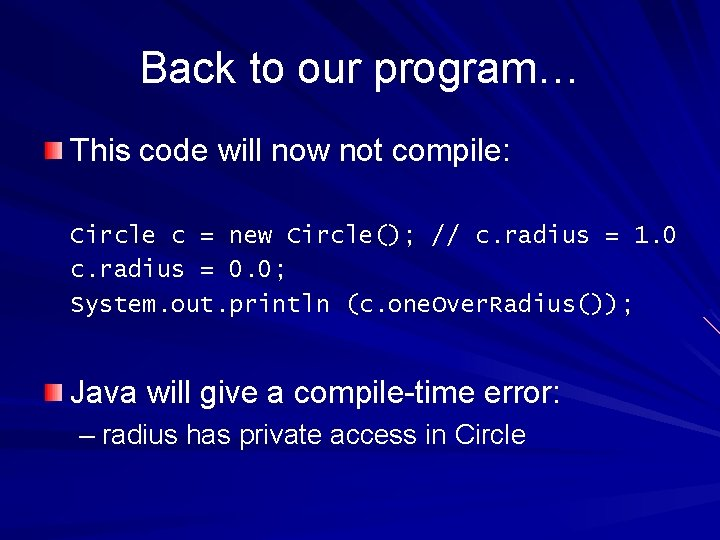Back to our program… This code will now not compile: Circle c = new Circle(); // c. radius = 1. 0 c. radius = 0. 0; System. out. println (c. one. Over. Radius()); Java will give a compile-time error: – radius has private access in CircleBack to our program… This code will also not compile: Circle c = new Circle(); // c. radius = 1. 0 System. out. println (c. radius); Java will give the same compile-time error: – radius has private access in Circle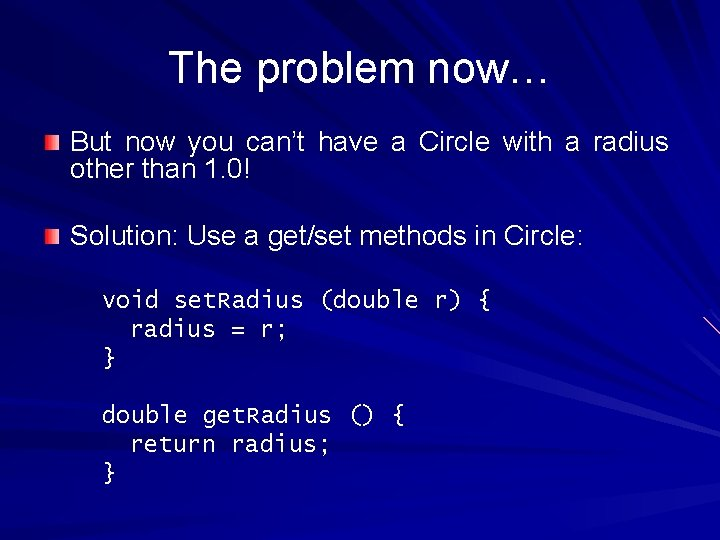The problem now… But now you can’t have a Circle with a radius other than 1. 0! Solution: Use a get/set methods in Circle: void set. Radius (double r) { radius = r; } double get. Radius () { return radius; }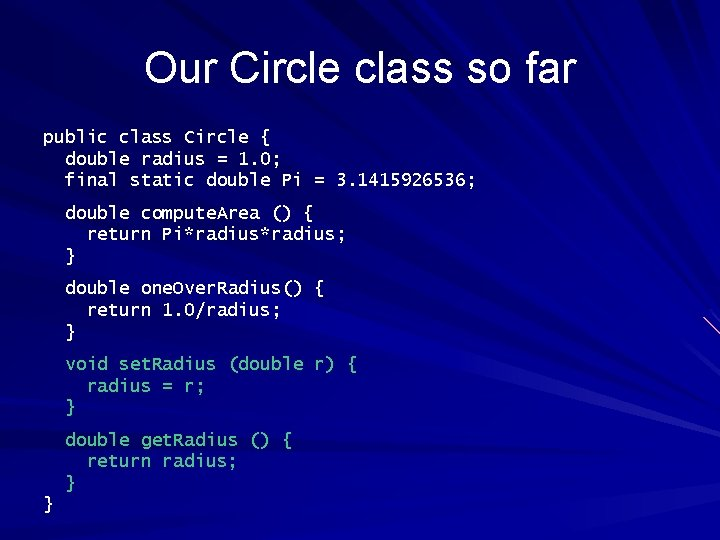Our Circle class so far public class Circle { double radius = 1. 0; final static double Pi = 3. 1415926536; double compute. Area () { return Pi*radius; } double one. Over. Radius() { return 1. 0/radius; } void set. Radius (double r) { radius = r; } double get. Radius () { return radius; } }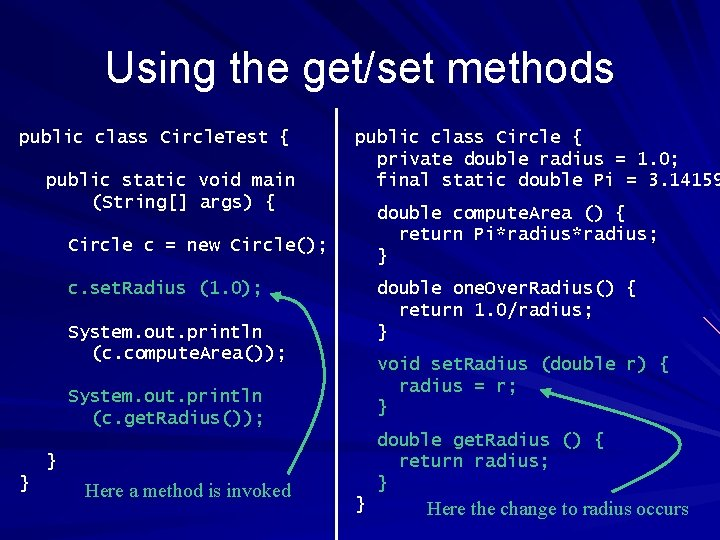Using the get/set methods public class Circle. Test { public static void main (String[] args) { public class Circle { private double radius = 1. 0; final static double Pi = 3. 14159 double compute. Area () { return Pi*radius; } Circle c = new Circle(); c. set. Radius (1. 0); double one. Over. Radius() { return 1. 0/radius; } System. out. println (c. compute. Area()); void set. Radius (double r) { radius = r; } System. out. println (c. get. Radius()); double get. Radius () { return radius; } } } Here a method is invoked } Here the change to radius occurs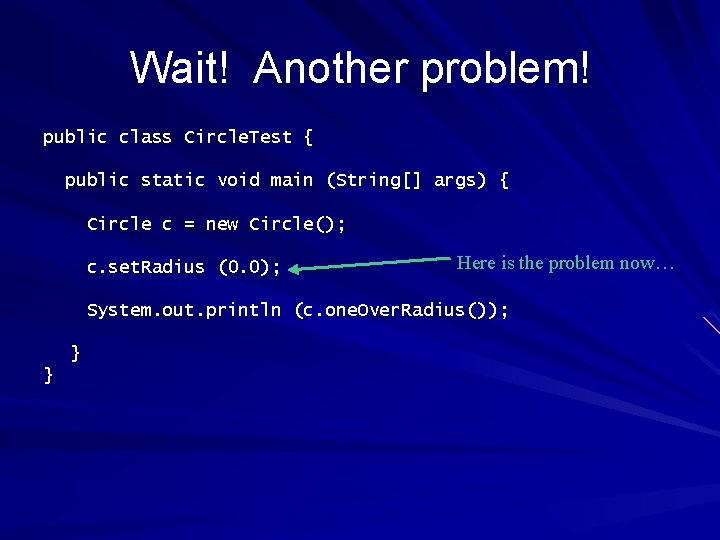Wait! Another problem! public class Circle. Test { public static void main (String[] args) { Circle c = new Circle(); c. set. Radius (0. 0); Here is the problem now… System. out. println (c. one. Over. Radius()); } }This problem is easily fixed Change the set. Radius method to the following void set. Radius (double r) { if ( r > 0. 0 ) radius = r; } Now there is no way for code outside the Circle class to change the radius to zeroVisibilities in Java There are four visibilities: – private: Only code within the same class can access the field or method Note: “access” means reading or writing the field, or invoking the method – public: Any code, anywhere, can access the field or method – protected: Used with inheritance We won’t get to that this semester – package: Almost the same as public This is the default Note that it can’t be specified like the othersA few notes on visibilities You can NOT specify visibilities for method variables – Any method variable can only be accessed within that method Think of it as public within the method (after it’s defined) and private outside the method You can also specify visibilities for methods and classes – More on that later in the course (or next course)Constructors When you create a object, you often have to do some initialization – You have to “construct” the object public class Circle { Note the different method declaration String name; public Circle() { Scanner scanner = new Scanner(System. in); System. out. println (“Enter the Circle’s name: ”); name = scanner. next(); } //. . . }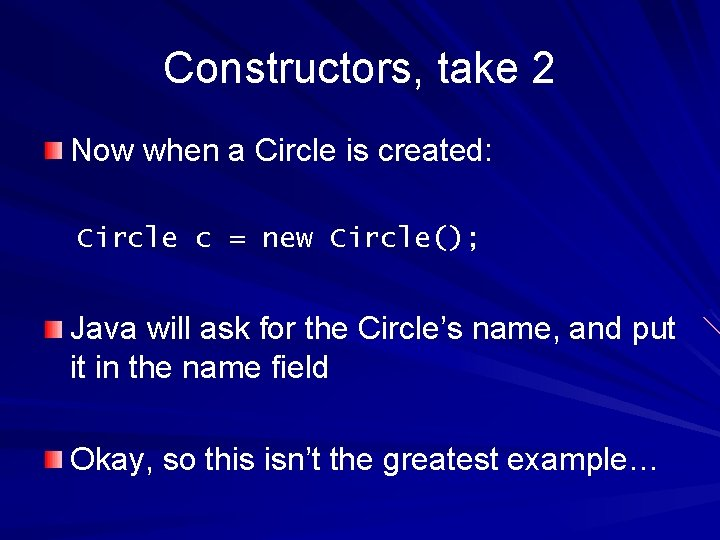Constructors, take 2 Now when a Circle is created: Circle c = new Circle(); Java will ask for the Circle’s name, and put it in the name field Okay, so this isn’t the greatest example…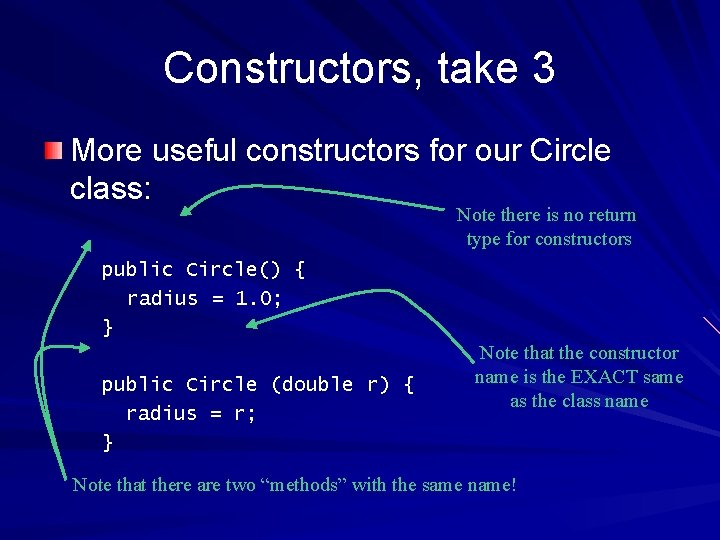Constructors, take 3 More useful constructors for our Circle class: Note there is no return type for constructors public Circle() { radius = 1. 0; } public Circle (double r) { radius = r; } Note that the constructor name is the EXACT same as the class name Note that there are two “methods” with the same name!Overriding methods (and constructors) Consider the following code: Circle c 1 = new Circle (); Circle c 2 = new Circle (2. 0); Creates a Circle of radius 1. 0 Creates a Circle of radius 2. 0 Java knows which constructor to call by the list of parameters – This is called “overloading”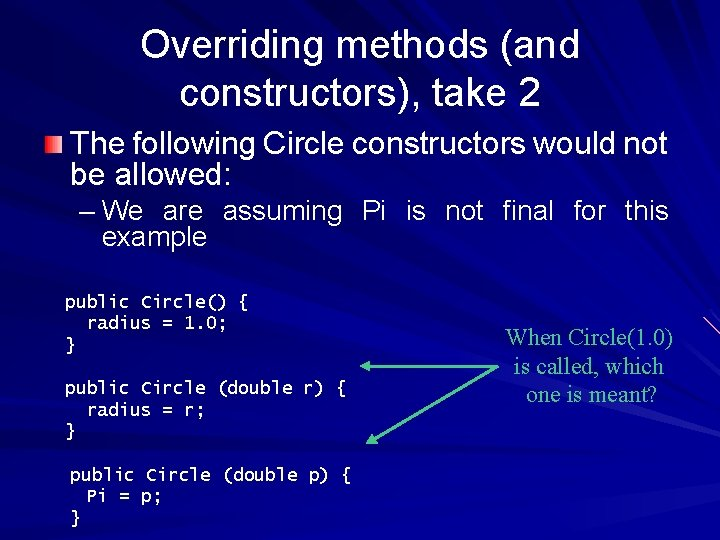Overriding methods (and constructors), take 2 The following Circle constructors would not be allowed: – We are assuming Pi is not final for this example public Circle() { radius = 1. 0; } public Circle (double r) { radius = r; } public Circle (double p) { Pi = p; } When Circle(1. 0) is called, which one is meant?Constructors, take 4 Our second constructor has a problem: public Circle (double r) { radius = r; } Consider the following code: Circle c = new Circle (0. 0); System. out. println (c. one. Over. Radius()); The method is dividing 1 by zero (again)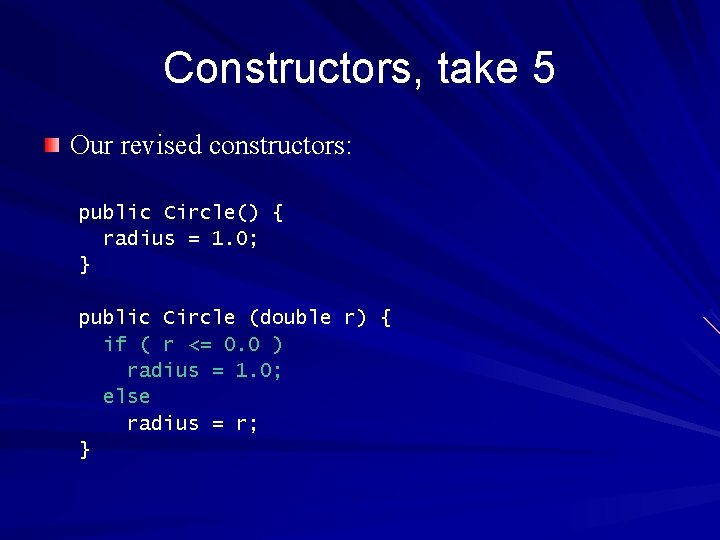Constructors, take 5 Our revised constructors: public Circle() { radius = 1. 0; } public Circle (double r) { if ( r <= 0. 0 ) radius = 1. 0; else radius = r; }A bit of humor…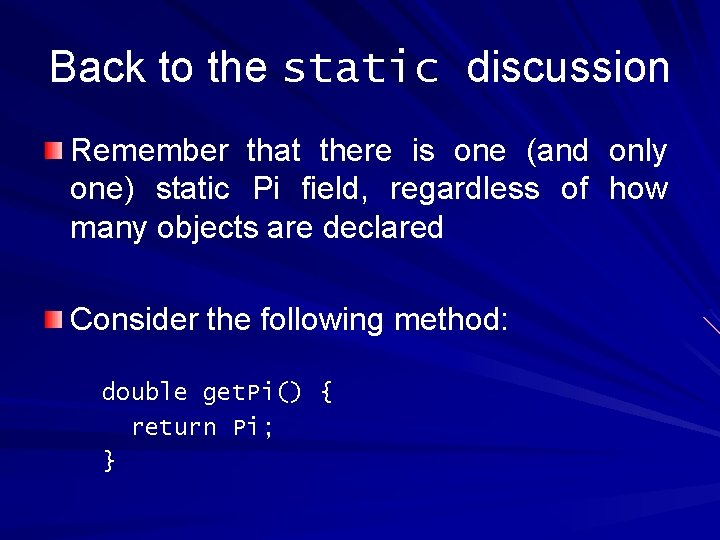Back to the static discussion Remember that there is one (and only one) static Pi field, regardless of how many objects are declared Consider the following method: double get. Pi() { return Pi; }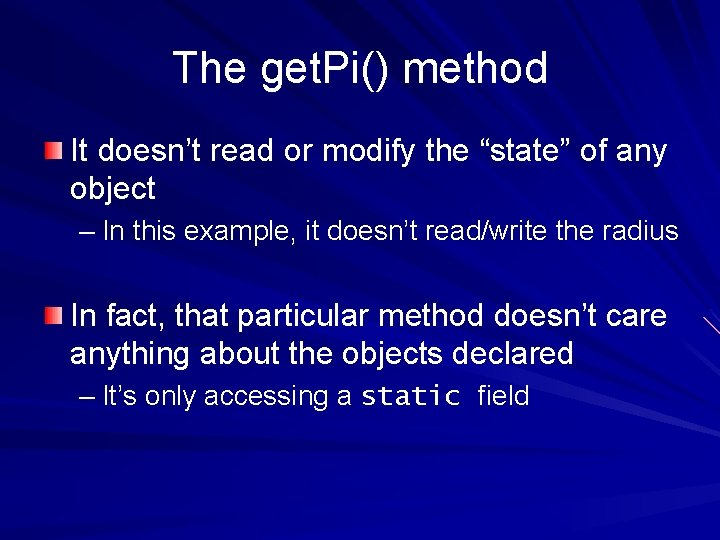The get. Pi() method It doesn’t read or modify the “state” of any object – In this example, it doesn’t read/write the radius In fact, that particular method doesn’t care anything about the objects declared – It’s only accessing a static field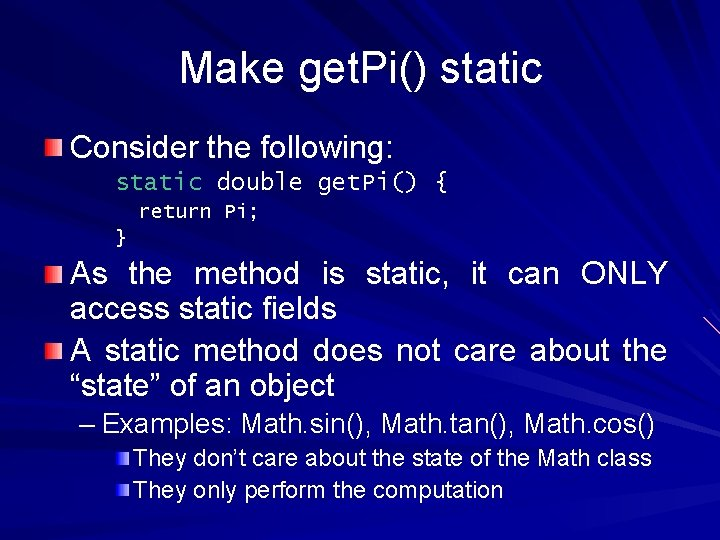Make get. Pi() static Consider the following: static double get. Pi() { return Pi; } As the method is static, it can ONLY access static fields A static method does not care about the “state” of an object – Examples: Math. sin(), Math. tan(), Math. cos() They don’t care about the state of the Math class They only perform the computationInvoking static methods As with static fields, they can be called using either an object or the class name: Circle c = new Circle(); System. out. println (c. get. Pi()); System. out. println (Circle. get. Pi());static methods and non-static fields Consider the following (illegal) Circle method: static double get. Radius() { return radius; } And the code to invoke it: public static void main (String[] args) { Circle c 1 = new Circle(); Circle c 2 = new Circle(); Circle c 3 = new Circle(); Circle c 4 = new Circle(); System. out. println (Circle. get. Radius()); }What happening in memory There are no 1 Circleobjects Circle objects memory in memory 4 million Circle ininmemory Which radius field does Circle. get. Radius() want? Circle c 1 Circle c 2 radius = 0. 0 … Circle c 3 radius = 0. 0 Pi = 3. 1415926536 Circle c 1000000 Circle c 4 radius = 0. 0The main static lesson A static method cannot access or modify the state of the objectstatic and non-static rules Non-static fields and methods can ONLY be accessed by the object name Static fields and methods can be accessed by Either the class name or the object name Non-static methods can refer to BOTH static and non-static fields Static methods can ONLY access static fields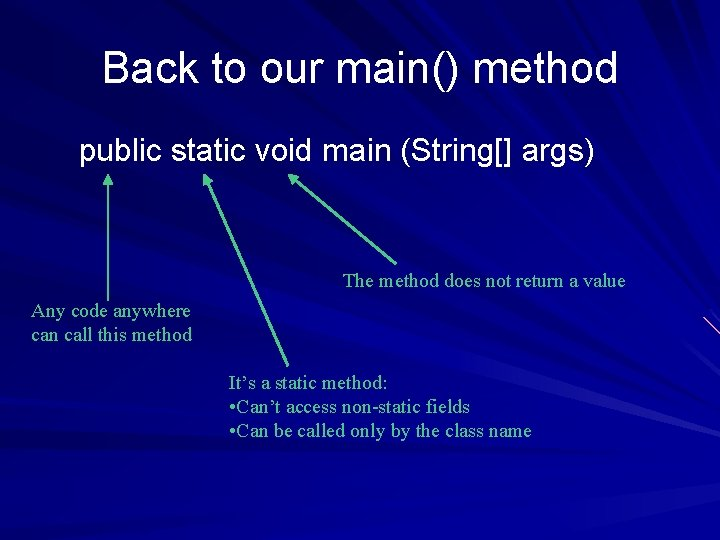Back to our main() method public static void main (String[] args) The method does not return a value Any code anywhere can call this method It’s a static method: • Can’t access non-static fields • Can be called only by the class nameA bit of humor…